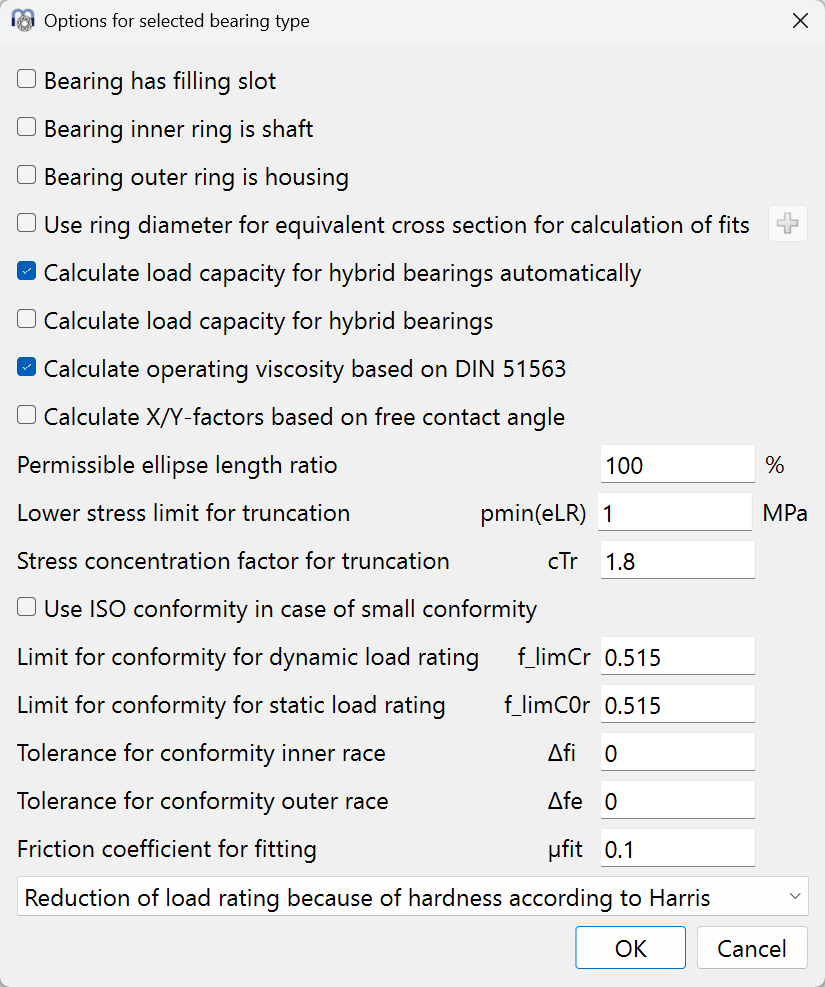﻿ Bearing types

# MESYS Calculation Software

 Bearing types

Different types of ball and roller bearings can be calculated using the software. In addition to the calculation of a single bearing, a configuration of several rows can be defined using “Bearing configuration”.By using the-button behind the bearing type, some options for the selected bearing type can be defined.

For all bearings, it can be selected that inner ring and shaft or outer ring and housing are identical. This affects the material input and the input of tolerances.

The option "Use ring diameter for equivalent cross section for calculation of fits" will lead to a larger ring thickness of inner and outer ring for the calculation of fits. Both variants can be compared using the graphic "Radial expansion of races". By using the-button behind the option, the race diameters can be overridden.

If the option "Calculate load capacity for hybrid bearings automatically" is active, then the next option is automatically set for hybrid bearing (Er >= 300 GPa, Ei, Ee < 260GPa)

The option “Calculate load capacity for hybrid bearings” will use (ISO 20056-1, 2017) and (ISO 20056-2, 2017) for the calculation of load capacities which leads to an increased static load capacity because of higher permissible stresses. The Youngs modulus of the rolling elements is required to be larger than 300GPa for the increased static permissible stress.

If the option "Calculate X/Y-factors based on free contact angle", then the effective free contact angle will be used to calculate the X/Y-factors in ISO 281 instead of the nominal contact angle. This will mainly affect deep groove ball bearings, where the free contact angle is increased with clearance.

For ball bearings the "Permissible ellipse length ratio" affects a warning about truncation and an output for a permissible axial force in the report.

For ball bearings the "Lower stress limit for truncation" ignores truncation for all contacts with a contact stress less than this limit.

For ball bearings the option "Use ISO conformity in case of small conformity" will use fi = fe = 0.52 for calculation of load ratings in case fi < 0.52 and fe < 0.53 for radial ball bearings and fi = fe = 0.535 if fi < 0.54 and fe < 0.54. These are the limits were tabulated values for fc in ISO 281 may be used.

For ball bearings, a limit for the conformity used in calculation of load capacity can be defined. The load capacity is calculated by the maximum value of the given limit and the actual geometry input. This input is only available in the above option regarding ISO conformity is not set.

For deep groove ball bearings a tolerance for the conformity can be defined. This will lead to additional outputs in the Tolerances Report.

If a friction coefficient for fitting is defined, an axial mounting force is shown in the tolerance report.

The reduction of the load capacities because of hardness can be calculated according to Harris or Schaeffler. The option according to Schaeffler leads to larger load capacities for very low hardness.

In the current version, the following bearing types are supported: